## Analogy

#### Analogy

Direction: Which one of the answer figures shall complete the given analogy?

1. Find the question mark ? figure from answer figure.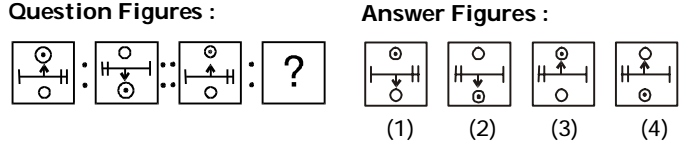1. From first figure to second figure both the circles interchange position, the large line segment is rotated through 180° and the arrow is inverted. IInd logic: Here 1 = 3 and 2 = 4 analogy will apply.

##### Correct Option: B

From first figure to second figure both the circles interchange position, the large line segment is rotated through 180° and the arrow is inverted. IInd logic: Here 1 = 3 and 2 = 4 analogy will apply.

1. Find the question mark ? figure from answer figure.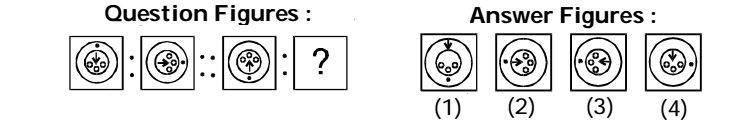1. The black dot is moving in clockwise direction while the designs of inner circle are moving in anticlockwise direction.

##### Correct Option: C

The black dot is moving in clockwise direction while the designs of inner circle are moving in anticlockwise direction.

1. Find the question mark ? figure from answer figure.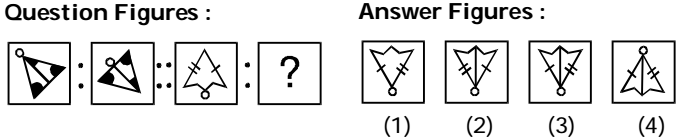1. From first figure to second figure the main design is rotated through 180° while the small circle shifts to opposite side.

##### Correct Option: C

From first figure to second figure the main design is rotated through 180° while the small circle shifts to opposite side.

1. Identify the two figures which are similar in all respects.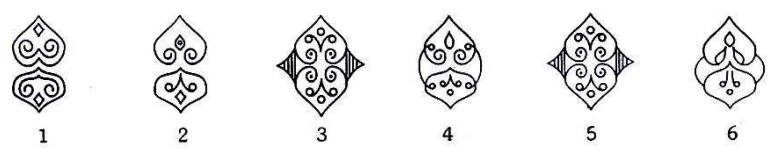1. Figures (3) and (5) are similar in all respects.

##### Correct Option: C

Figures (3) and (5) are similar in all respects.

1. Four pairs of flower pots are given below. Among them only one pair is similar in all respects. Identify the pair numbers which represent that pair.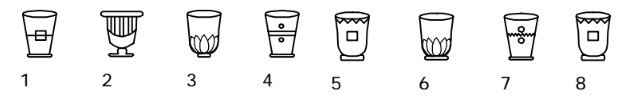1. Figures (5) and 8 are similar.

##### Correct Option: C

Figures (5) and 8 are similar.